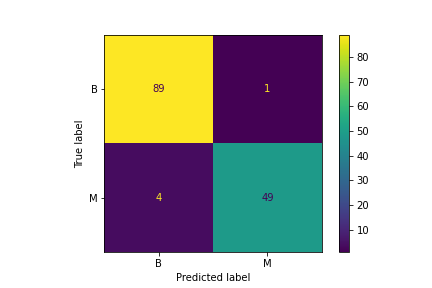# Model description

This is a Logistic Regression trained on breast cancer dataset.

## Intended uses & limitations

This model is trained for educational purposes.

## Training Procedure

### Hyperparameters

The model is trained with below hyperparameters.

Click to expand
Hyperparameter Value
memory
steps [('scaler', StandardScaler()), ('model', LogisticRegression())]
verbose False
scaler StandardScaler()
model LogisticRegression()
scaler__copy True
scaler__with_mean True
scaler__with_std True
model__C 1.0
model__class_weight
model__dual False
model__fit_intercept True
model__intercept_scaling 1
model__l1_ratio
model__max_iter 100
model__multi_class auto
model__n_jobs
model__penalty l2
model__random_state
model__solver lbfgs
model__tol 0.0001
model__verbose 0
model__warm_start False

### Model Plot

The model plot is below.

Pipeline(steps=[('scaler', StandardScaler()), ('model', LogisticRegression())])
Please rerun this cell to show the HTML repr or trust the notebook.

## Evaluation Results

You can find the details about evaluation process and the evaluation results.

Metric Value
accuracy 0.965035
f1 score 0.965035

# How to Get Started with the Model

Use the code below to get started with the model.

import joblib
import json
import pandas as pd
with open("config.json") as f:
clf.predict(pd.DataFrame.from_dict(config["sklearn"]["example_input"]))